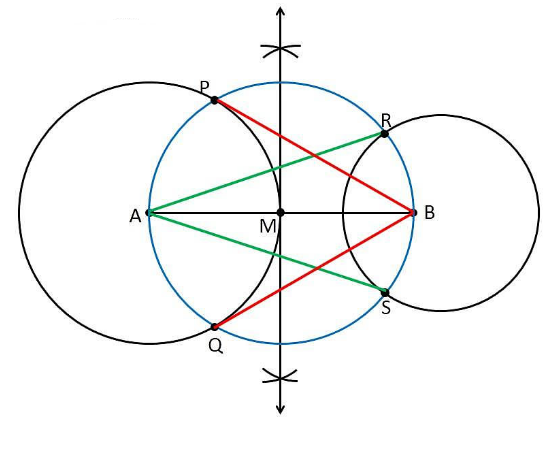# Draw a line segment AB of length 8 cm. Taking A as centre, draw a circle of radius 4 cm and taking B as centre, draw another circle of radius 3 cm. Construct tangents to each circle from the centre of the other circle. Give the justification of the construction

To construct a tangent from given conditions

1. Let us draw a line segment AB = 8 cm.

2. Considering A as a centre and draw a circle of radius 4 cm

3.Considering B as the centre, draw a circle of radius 3 cm

4. Draw the perpendicular bisector of the line AB and the midpoint is taken as M.

5. Now, take M as the centre draw a circle with the radius of MA or MB which intersects the circle at the points P, Q, R and S.

6. Now join AR, AS, BP and BQ

7. Therefore, the required tangents are AR, AS, BP and BQJustification

We have to prove that AS and AR are the tangents of the circle (whose centre is B with radius is 3 cm) and BP and BQ are the tangents of the circle (whose centre is A and radius is 4 cm).

From the construction, to prove this,

let us join AP, AQ, BS, and BR.

∠ASB is an angle in the semi-circle. We know that an angle in a semi-circle is a right angle.

∴ ∠ASB = 90°

⇒ BS ⊥ AS

Since BS is the radius of the circle, AS must be a tangent of the circle.

Similarly, AR, BP, and BQ are the required tangents of the given circle.